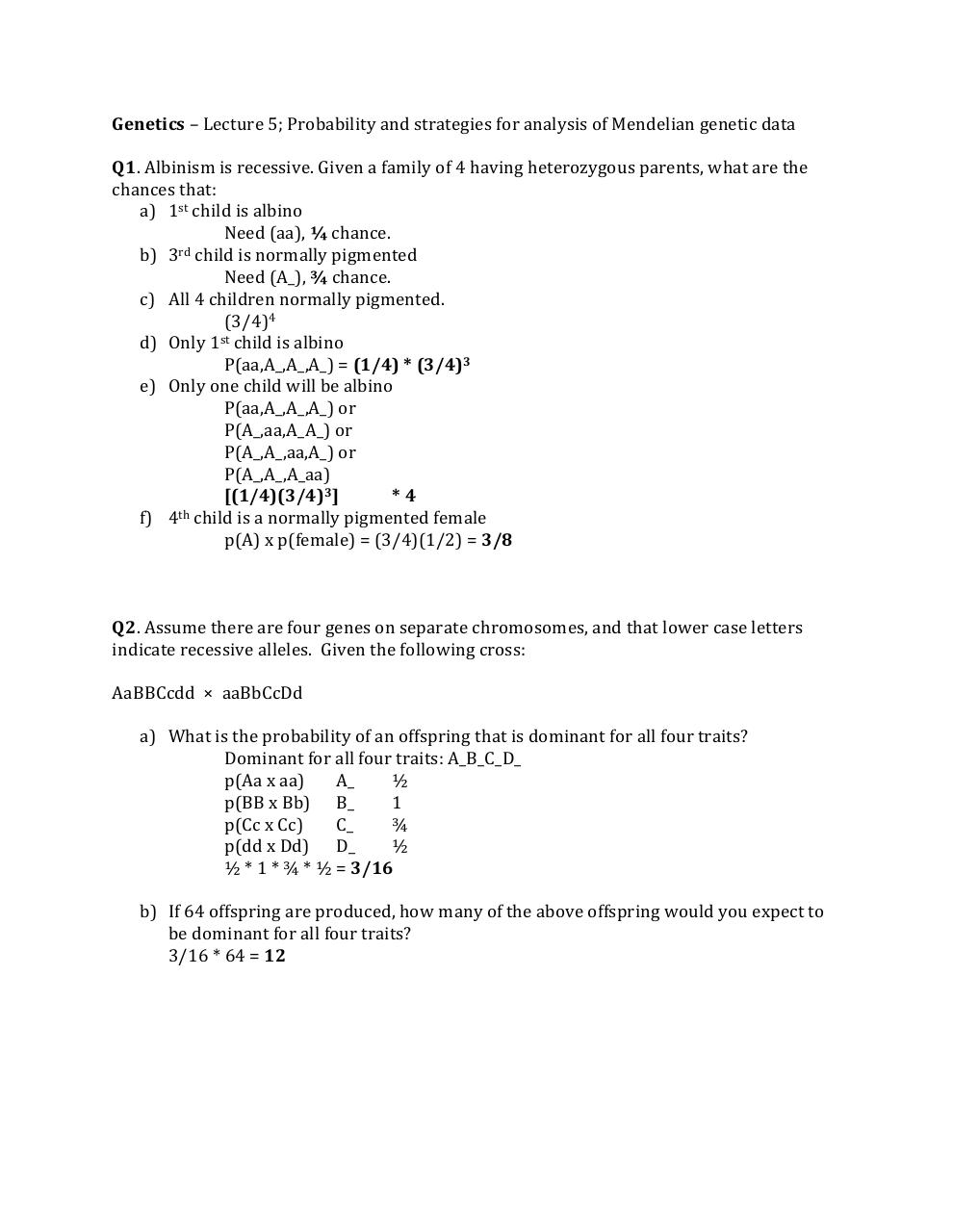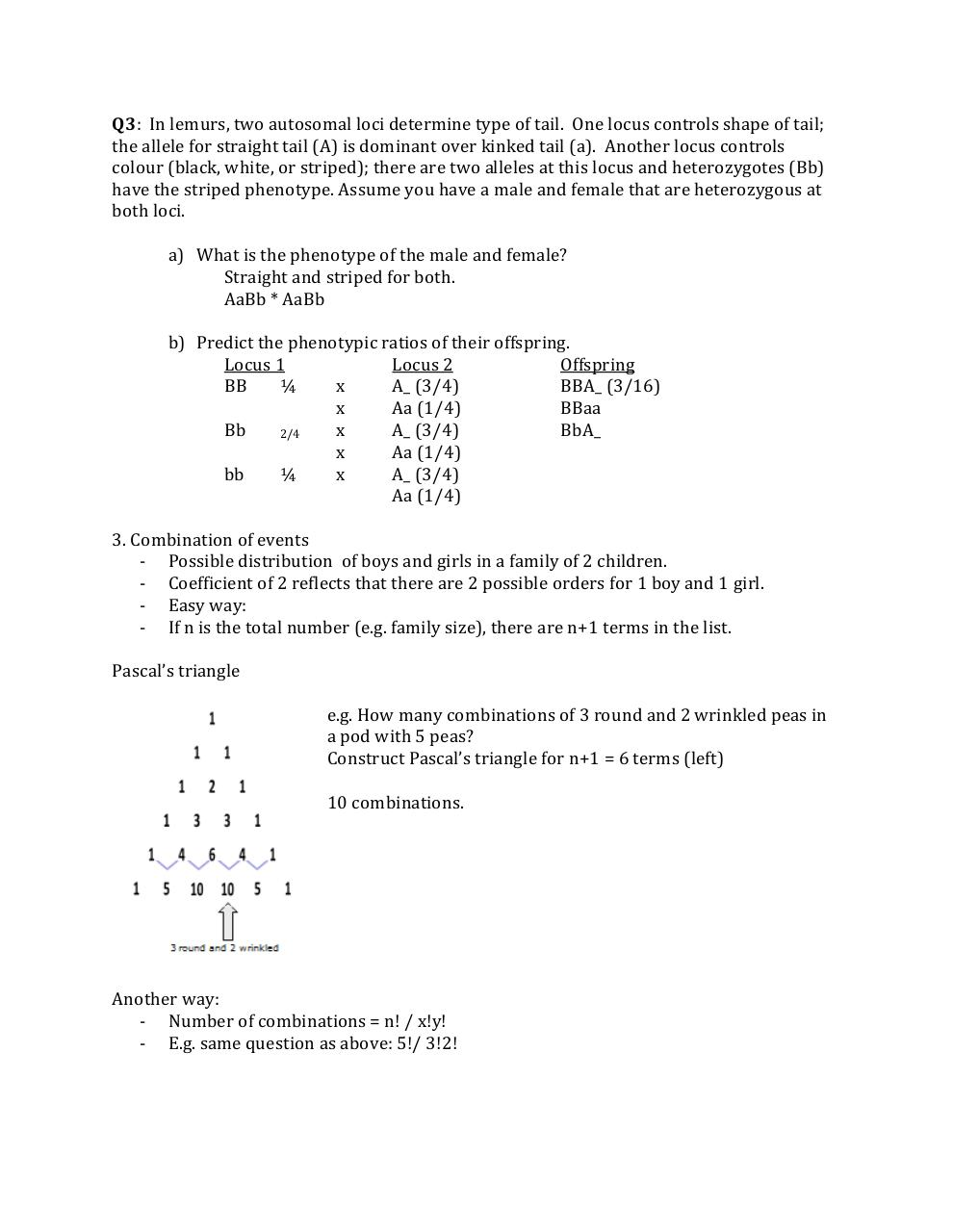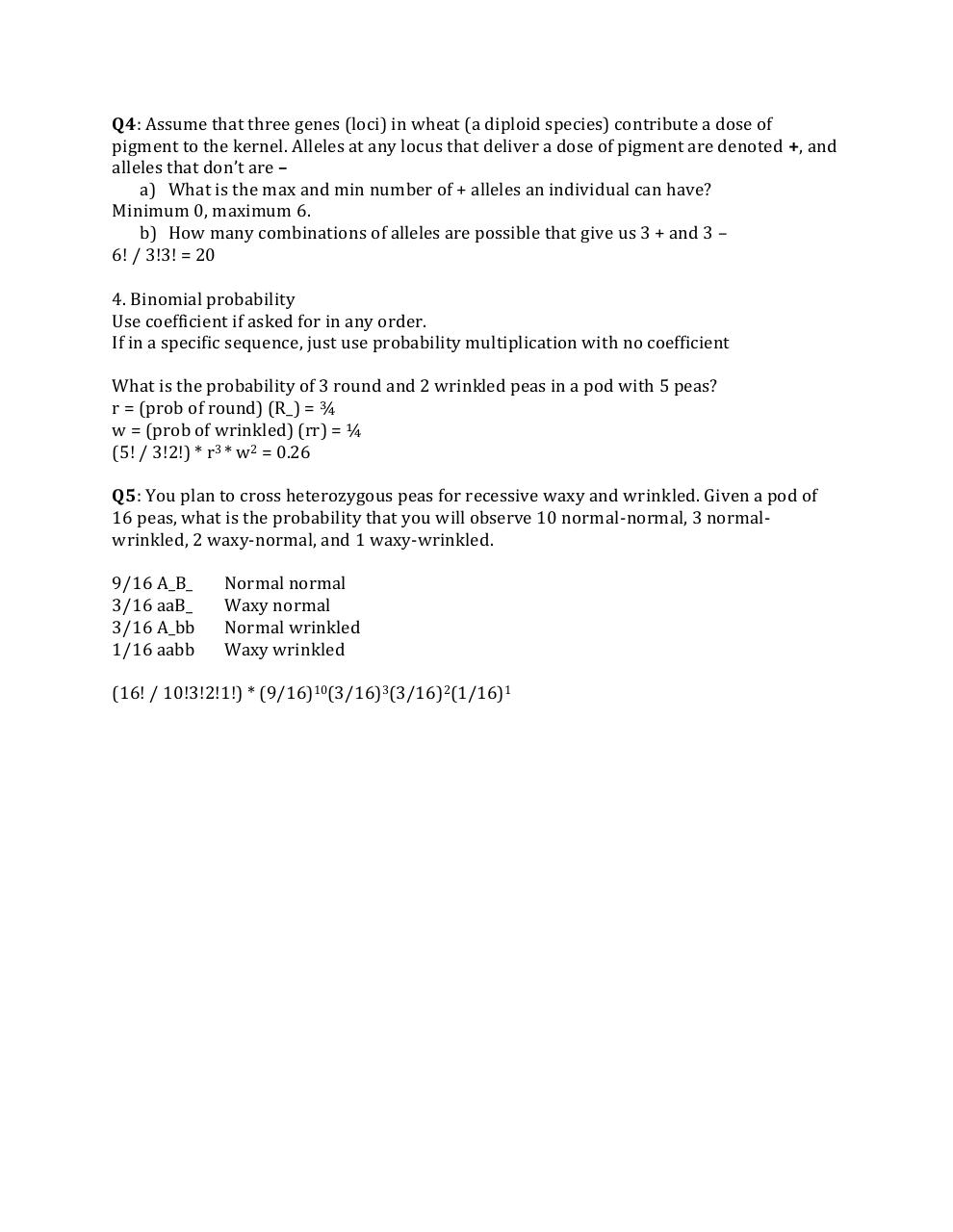# Notes Lecture 5; Strategies for analysis .pdf

### File information

Original filename: Notes - Lecture 5; Strategies for analysis.pdf
Author: Chris

This PDF 1.5 document has been generated by MicrosoftÂ® Office Word 2007, and has been sent on pdf-archive.com on 05/02/2015 at 00:48, from IP address 142.68.x.x. The current document download page has been viewed 730 times.
File size: 412 KB (3 pages).
Privacy: public file

Notes - Lecture 5; Strategies for analysis.pdf (PDF, 412 KB)

### Document preview

Genetics – Lecture 5; Probability and strategies for analysis of Mendelian genetic data
Q1. Albinism is recessive. Given a family of 4 having heterozygous parents, what are the
chances that:
a) 1st child is albino
Need (aa), ¼ chance.
b) 3rd child is normally pigmented
Need (A_), ¾ chance.
c) All 4 children normally pigmented.
(3/4)4
d) Only 1st child is albino
P(aa,A_,A_,A_) = (1/4) * (3/4)3
e) Only one child will be albino
P(aa,A_,A_,A_) or
P(A_,aa,A_A_) or
P(A_,A_,aa,A_) or
P(A_,A_,A_aa)
[(1/4)(3/4)3]
*4
th
f) 4 child is a normally pigmented female
p(A) x p(female) = (3/4)(1/2) = 3/8

Q2. Assume there are four genes on separate chromosomes, and that lower case letters
indicate recessive alleles. Given the following cross:
AaBBCcdd × aaBbCcDd
a) What is the probability of an offspring that is dominant for all four traits?
Dominant for all four traits: A_B_C_D_
p(Aa x aa)
A_
½
p(BB x Bb) B_
1
p(Cc x Cc)
C_
¾
p(dd x Dd) D_
½
½ * 1 * ¾ * ½ = 3/16
b) If 64 offspring are produced, how many of the above offspring would you expect to
be dominant for all four traits?
3/16 * 64 = 12

Q3: In lemurs, two autosomal loci determine type of tail. One locus controls shape of tail;
the allele for straight tail (A) is dominant over kinked tail (a). Another locus controls
colour (black, white, or striped); there are two alleles at this locus and heterozygotes (Bb)
have the striped phenotype. Assume you have a male and female that are heterozygous at
both loci.
a) What is the phenotype of the male and female?
Straight and striped for both.
AaBb * AaBb
b) Predict the phenotypic ratios of their offspring.
Locus 1
Locus 2
Offspring
BB
¼
x
A_ (3/4)
BBA_ (3/16)
x
Aa (1/4)
BBaa
Bb
x
A_ (3/4)
BbA_
2/4
x
Aa (1/4)
bb
¼
x
A_ (3/4)
Aa (1/4)
3. Combination of events
- Possible distribution of boys and girls in a family of 2 children.
- Coefficient of 2 reflects that there are 2 possible orders for 1 boy and 1 girl.
- Easy way:
- If n is the total number (e.g. family size), there are n+1 terms in the list.
Pascal’s triangle
e.g. How many combinations of 3 round and 2 wrinkled peas in
a pod with 5 peas?
Construct Pascal’s triangle for n+1 = 6 terms (left)
10 combinations.

Another way:
- Number of combinations = n! / x!y!
- E.g. same question as above: 5!/ 3!2!

Q4: Assume that three genes (loci) in wheat (a diploid species) contribute a dose of
pigment to the kernel. Alleles at any locus that deliver a dose of pigment are denoted +, and
alleles that don’t are –
a) What is the max and min number of + alleles an individual can have?
Minimum 0, maximum 6.
b) How many combinations of alleles are possible that give us 3 + and 3 –
6! / 3!3! = 20
4. Binomial probability
Use coefficient if asked for in any order.
If in a specific sequence, just use probability multiplication with no coefficient
What is the probability of 3 round and 2 wrinkled peas in a pod with 5 peas?
r = (prob of round) (R_) = ¾
w = (prob of wrinkled) (rr) = ¼
(5! / 3!2!) * r3 * w2 = 0.26
Q5: You plan to cross heterozygous peas for recessive waxy and wrinkled. Given a pod of
16 peas, what is the probability that you will observe 10 normal-normal, 3 normalwrinkled, 2 waxy-normal, and 1 waxy-wrinkled.
9/16 A_B_
3/16 aaB_
3/16 A_bb
1/16 aabb

Normal normal
Waxy normal
Normal wrinkled
Waxy wrinkled

(16! / 10!3!2!1!) * (9/16)10(3/16)3(3/16)2(1/16)1#### HTML Code

Copy the following HTML code to share your document on a Website or Blog

#### QR Code### Related keywords